# GMAT Probability | 3 deadly mistakes to avoid | GMAT Quant

When it comes to GMAT Quant, Probability is like the ‘iceberg’ with which the Titanic collided, and the ‘so-called’ unsinkable ship, sunk. Falling in GMAT Probability pitfalls can be hazardous to your GMAT score. Although, probability is a simple concept, yet its application in GMAT ends up hitting your score. That is why we have come up with this article which will help you avoid the three deadly mistakes in GMAT probability.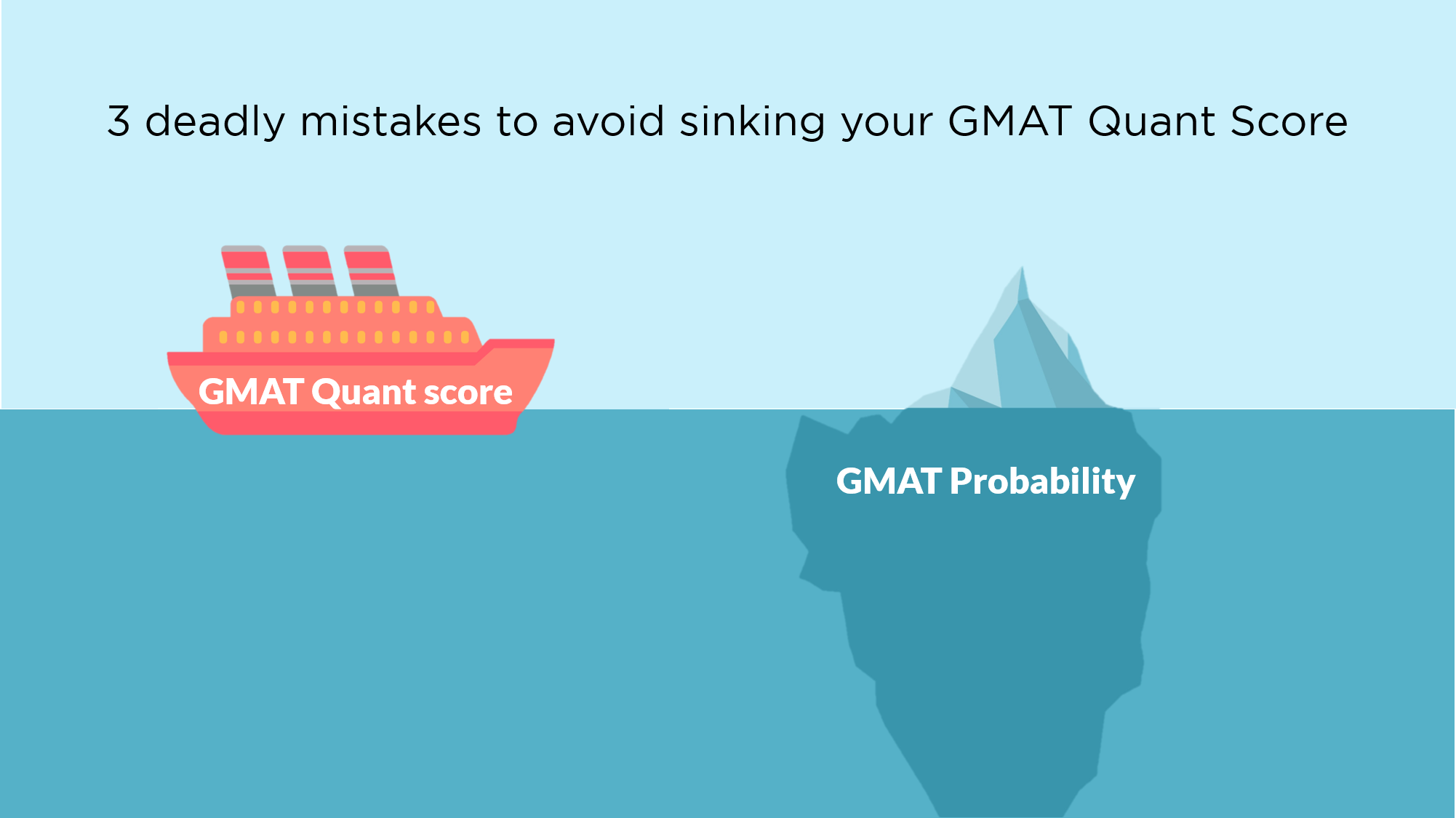Here is a brief outline of the sections covered in this article:

If you haven’t gone through our article series on Permutation and Combination, we suggest reading it first.

• Are you someone who doesn’t know how to solve a GMAT probability question?
• Are you confused which cases should be considered and which cases should be ignored while calculating the probability?
• Are you someone who is confused while considering all the cases of arrangement?

If the answer to any of the above questions is yes, then we strongly recommend you continue reading the article.

In this article, you will learn a structured approach to solve probability questions. We will also explain 3 most common mistakes that students make in GMAT probability questions and the best way to solve such questions.

In the article, we will learn:

• How to solve a probability question by the non-event method
• How to calculate the probability when more than 1 arrangement is possible
• How to find the greatest or lowest value of the probability

Now let us begin the article with a very frequently used concept of non-events in probability.

Wondering what are necessary but not sufficient conditions in GMAT Verbal? Read the article to learn more.

## Non-Event method for solving GMAT Probability Questions

At times, we may come across a GMAT probability question, in which we need to find a lot of favorable cases, which is known as favorable events in probability jargon. Now, finding all the cases is time-consuming and might involve a lot of calculations. In such situations, we may end up making a mistake too.

So, how should we tackle such a situation? Obviously, there has to be a better way to do it! Well, the better way or better method is known as the – Non-Event Method, in which we find all the cases which we do not want (known as non-event) and subtract it from the favorable cases.

This method reduces a lot a calculation but at the same place, test your logical thinking skills.

Let’s understand what I am trying to say with the help of an example!

### Q -If x is to be chosen at random from the set {1,2,3,4} and y is to be chosen at random from the set {5,6,7}, what is the probability that x × y will be even?

Solution

Now, how should be we solve this question?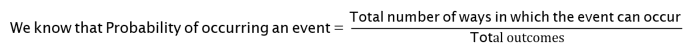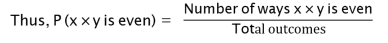Thus, we need two things:

Number of ways x × y is even Total outcomes

• The number of ways in which the multiplication of x and y is an even number and,
• Total outcomes we can get when we take all possible combinations of x and y and multiply them with each other

Now, we can easily calculate the above two by applying the concepts of permutation and combination.

Number of ways in which x × y is even:

x × y can be even in three ways:

1. When both, x and y, are even Or,
2. When x is even, and y is odd Or,
3. When x is odd, and y is even

Thus,

Total ways = (Ways in which both x and y are even) + (Ways in which only x is even) + (Ways in which only y is even)

• Total ways = 2C1 × 1C1 + 2C1 × 2C1 + 2C1 × 1C1 = 2 + 4 + 2 = 8

Total outcomes

Total outcomes= Ways in which x can be selected * Ways in which y can be selected

• Total outcomes = 4 × 3 = 12

Thus, P (x × y is even) = (8/12) = (2/3)
Although this example was an easy one and we got 3 cases, what if the first set has 8 numbers and the second set had 9 numbers?

Then counting every possible case will be tedious.

Let us now solve this question with another approach – Non-event method.

Non-event Method:

The multiplication of x and y can give only two possibilities:

• Either x × y is even or,
• x × y is odd

Thus, if we add the probabilities of all the possible cases, then their sum should be equal to 1. Hence,

• P (x × y is even) + P (x × y is odd) = 1 or,
• P (x × y is even) = 1 – P (x × y is odd)

Thus, if we find P (when x × y is odd) then we just need to subtract its values from 1 to get the actual answer.

And, surprisingly the number of ways when x × y is odd is only 1- When x and y both are odd!!! Total ways when x × y is odd = Ways when x is odd × Ways when y is odd = 2C1 × 2C1= 4

Thus, P (x × y is even) = [1-(4/12)] = 8/12

Oh!! This was easy!

So, can you observe that you have to think a step ahead to visualize which method will be easy for you? And yes, non-event method reduced our calculation too.

Thus, learning from the above example is that whenever we see that there are a lot of favorable events, we should always check if the non-events is less. And if that is the case, we should find the non-events first and then subtract it from the total cases.

Now, let’s come to come to the common type of errors that a student makes while solving the questions by non-event method.

Want to ace GMAT Critical Reasoning. Here is a video lesson.

## Deadly Mistake 1 – Missing cases

Let us take an interesting example. Try to solve the question first before moving to the solution. There is a high possibility that you might end up making the mistake highlighted in the later part of the article.

### Q – In a box of 12 pens, a total of 3 are defective. If a customer buys 2 pens selected at random from the box., what is the probability that neither pen will be defective?

Solve this question by non-event method only.

Solution

Do you know, how I already knew you arrived at 21/22?

You must have solved using the below formula

P (neither pen will be defective) = 1- P (Both the pens are defective)?

If that is the case, then you made a very common error!!! You missed a few cases. Let us see how to correctly apply the non-event method in this question

Correct approach:

Let us write all the possible cases in which customer can buy the two pens.

• He can get both the non-defective pens Or,
• He can get 1 defective pen and 1 non-defective pen Or
• He can get both the defective pen

Since the sum of the probability of all the possible cases is 1, thus

• P (neither pen is defective) + P (1 defective pen and 1 non-defective pen) + P (Both the pens are defective) = 1

Now, can you find P (neither pen is defective)?

• Thus, P (neither pen is defective) = 1 – P (Both the pens are defective) – P (1 defective pen and 1 non-defective pen)

Can you see the mistake you made when you solved using the formula? P (neither pen will be defective) = 1- P (Both the pens are defective)?

• You missed one case. This is an error of Missing cases.

Now, we only have to calculate the values of P (None of the pens is defective) and P (1 defective pen and 1 non-defective pen), and we will have the answer.

But to arrive at the above equation of P (Both the pens are defective), you need to think logically, since GMAT tests your logical thinking skills.

Now, let us find P (Both the pens are defective) and P (1 defective pen and 1 non-defective pen).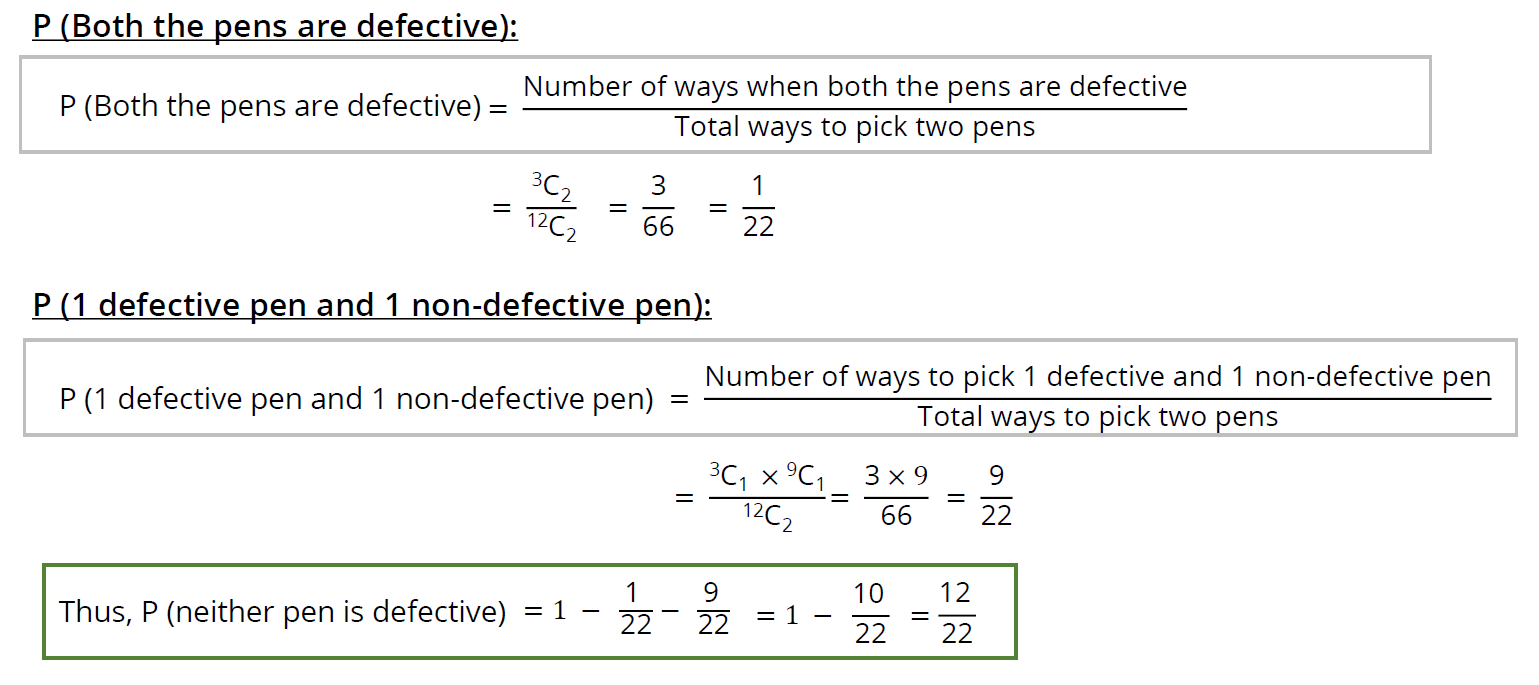Takeaways

1. Before solving GMAT probability questions by the non-event method, you should come up with all the possible cases.
2. Once you write the sum of probabilities of all the possible cases equal to 1, you can easily find the answer by non-event method.

## Deadly Mistake 2 – Considering arrangement

While solving any GMAT probability question, most of the students forget that for different arrangements of a particular case, the probability is also different. They do not consider all the arrangements and calculate the probability of one of the possible cases which results in the wrong answer.

Let us understand what I am trying to say with the help of an example.

### Q – There are 10 solid-colored balls in a box including 1 green ball and 1 yellow ball. If 3 of the balls in the box are to be chosen at random without replacement, what is the probability that three balls chosen will include the green ball but not the yellow ball?

Solution:

Now, have you started thinking of all the possible cases?

If not, do not worry!!! After reading this article and practicing questions, thinking of all the possible cases will be the next step you will be doing without even realizing.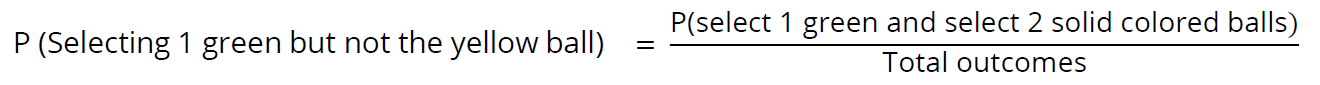Thus,

• Probability = P (selection of 1 green ball) × P (selecting 1st solid ball) × P (Selecting 2nd solid ball)
• We can select 1 green from 10 balls in only 1 way thus,
• P (selection of 1 green ball) = 1/10
• Now, we have 9 remaining balls. Thus,
• P (selecting 1st solid ball) = 8/9 and,
• P (Selecting 2nd solid ball) = 7/8

Hence, P (Selecting 1 green but not the yellow ball) = (1/10) × (8/9) × (7/8) = (7/90)

No!!!!! it is not the final answer. But most of the students will mark 7/90 as the correct answer to this question.

Let us understand why this answer is not correct.

Can you find in how many ways we can choose 3 balls such that 1 ball is green, and 2 balls are solid colored???

Let us list down the possible orders.

• G S S
• S G S
• S S G

Total cases = 3

We can also think in this way that we have balls- 1 green and 2 Solid colored balls. In how many we can arrange them?

This is similar to the case of identical objects. Thus,

Total ways = 3!/2! = 3

So, there are 3 arrangements possible, but we considered only 1 case i.e G S S and calculated its probability.

• Should we add the probabilities of all the different cases?
• Okay, this is a good way to But what if we have a number larger than 3???
• Let’s suppose out of 15 cases we considered only 1 case then how will you find the answer???
• Will you add the probabilities of all the Cases?
• No, right!!

So, let us look at another way!

Let us first find P(S G S) and P(S S G).

• P(S G S) = (8/10) x (1/9) x (7/8) = 7/90 = P(G S S)
• P(G S S) = (8/10) x (1/9) x (7/8) = 7/90 = P(G S S)

If you observe, then all the three cases have the same probability. Thus, multiplying 3 with P(G S S) will give our final answer.

And 3 is the number of ways in which you can select 3 balls – 1 green and 2 Solid colored balls.

Takeaways

If we replace 3 by the number of ways balls can be arranged, then we can conclude:

1. If there are more than one arrangements possible, then we will find the probability of only one case and multiply it by the total number of possible cases. This will give us our final answer.
2. The first step to solve every probability question is to think of all the possible cases.

### Q – A box contains 100 balls, numbered from 1 to 100. If three balls are selected at random and with replacement from the box, what is the probability that the sum of the three numbers on the three balls selected will be odd?

Solution:

Can you think of all the possible cases in which we can get the sum of three numbers to be odd? There are 2 possible cases:

• When all the three numbers are odd or,
• When two numbers are even, and one is

Probability when all the numbers are odd:

• = P(Odd) × P(Odd) × P(Odd)
• = (1/2) x (1/2) x (1/2) = (1/8)

Probability when two numbers are even, and one is odd:

• = P(Odd) × P(Even) × P(Even)
• =(1/2) x (1/2) x (1/2) = (1/8)

However, this case can occur in 3!/2! = 3 ways

• Odd, Even, Even
• Even, Odd, Even
• Even, Even, Odd

Hence,

P(two numbers are even, and one is odd) = 3 × (1/8)

Thus, total probability = (1/8) + (3/8) = (4/8) = 1/2

## Deadly Mistake 3 – Confusing dependent and independent events

Let us look at a slightly complex problem of GMAT probability and a question that is put so beautifully that most of the aspirants fall prey to the trap of this question and mark a wrong answer.

Do you want to test your knowledge of probability? Let’s give it a try.

### Q – If the probability is 0.54 that stock A will increase in value during the next month and the probability is 0.68 that stock B will increase in value during the next month, what is the greatest value for the probability that neither of these two events will occur.

Is the answer equal to 0.1472 or 0.32?

Solution

Can you observe that the language of this question is a little different???

• In every question, we are asked to find what the probability that an event can occur, however in this question, we are asked to find the greatest probability such that both the events do not occur.
• So how should we find this?

We know how to find the probability but what is the greatest probability?

Well, don’t worry!! This is a question of probability where two events are dependent.

Let us understand independent and dependent events first.

Independent Events:

• We are tossing a coin two times
• Can we say that the occurrence of head/tail in the 2nd toss is dependent on the occurrence of head/tail in the first toss?
• No, right! It does not matter what we get in the first toss. It won’t affect toss 2.
• This is an example of Independent events

Dependent Events:

• Now, from the real-life experience, we all know that the prices of some stocks may be dependent on each other
• If the price of one stock increases, then the price of some stocks may increase, and the price of some other stocks may decrease
• If the price of the raw material decreases, then the price of stocks of a company that uses that raw material will increase as the company will make more gain now
• These events are dependent events

And our question is a perfect example of dependent events.

So, how do we calculate the greatest probability such that neither of these two events occur? Let us see two dependent events, A and B, pictorially.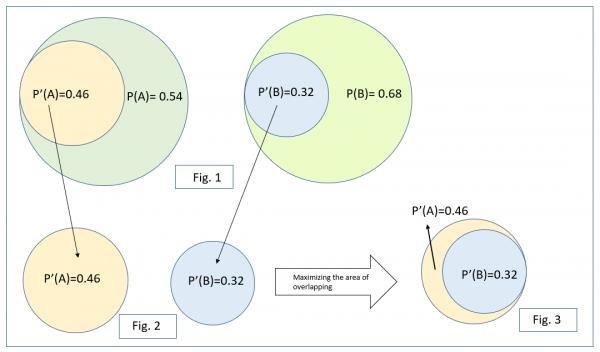Since P(A) = 0.54, P’(A) = 0.46

Here, the full circle represents the total probability in which all the events can occur (A might increase and decrease) and the small circle inside it represents the probability of all the events in which A can increase.

From the diagram, we know that we need to maximize the area/probability of P’(A).

So, how can we maximize this keeping in mind that A and B are dependent???

• How can we make the overlapping of event A’ and B’ greatest?
• This is shown in the diagram (Fig 2 and Fig.3)
• Fig.2 shows the areas of A’ and B’ and now we need to figure out the maximum overlap between these two.
• And we can do so, as shown in 3
• The complete overlap of event A’ with event B’ happens:
• when we map B’ inside A’ and “assume that when B does not increase A will also not increase at the same time.
• Hence, the probability of area such that both A and B does not occur= 0.32
• And this is the greatest probability because if we move B a little, the probability, 0.32, will reduce.
• Hence, our answer is 0.32.

For strategic advice and guidance about GMAT preparation email us at acethegmat@e-gmat.com.

Want to check if your GMAT Quant preparation is enough for a good GMAT score? Attend our free Quant Workshop and get personalised feedback and insights from Subject Matter Experts to reach your target Quant score.

This is the way to solve questions of dependent probability.

So, we learned 3 ways to avoid mistakes while solving probability questions. Let us summarize everything.

## Takeaways | GMAT Probability

1. The first step to solve any probability question is to think of all the possible cases.
2. To make sure that you are not missing any cases when solving by non-event method, write the sum of probabilities of all the possible cases equal to 1 then you can easily find the answer by non-event method.
3. If there is more than one arrangement possible then we find the probability of only one case and multiply by the total number of possible arrangements.
4. Always keep an eye on the keywords like- greatest probability and minimum probability and make sure to draw diagrams to visualize the overlap between the probabilities of 2 events.Payal Tandon